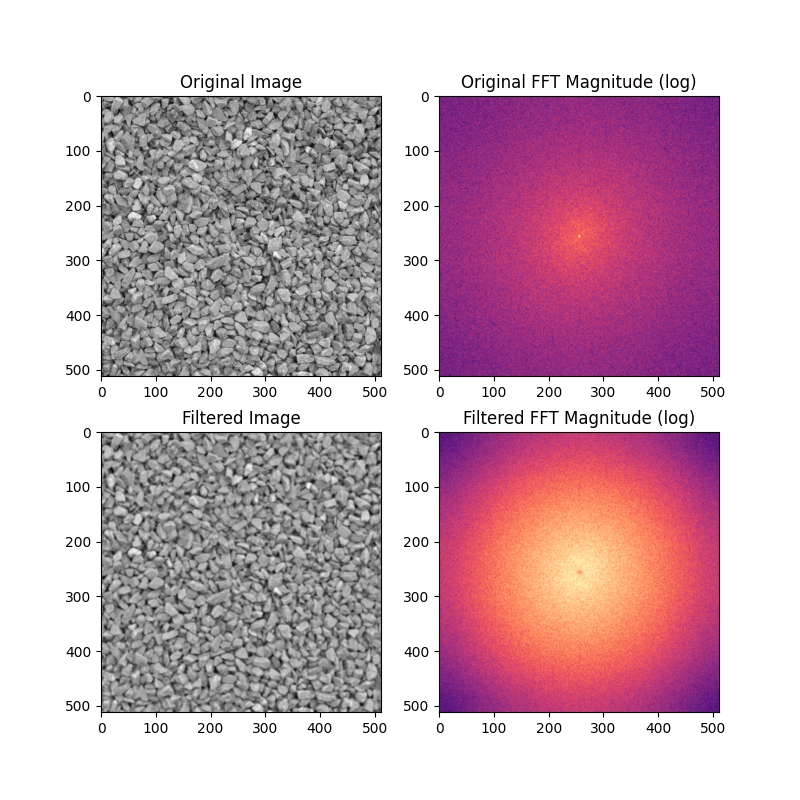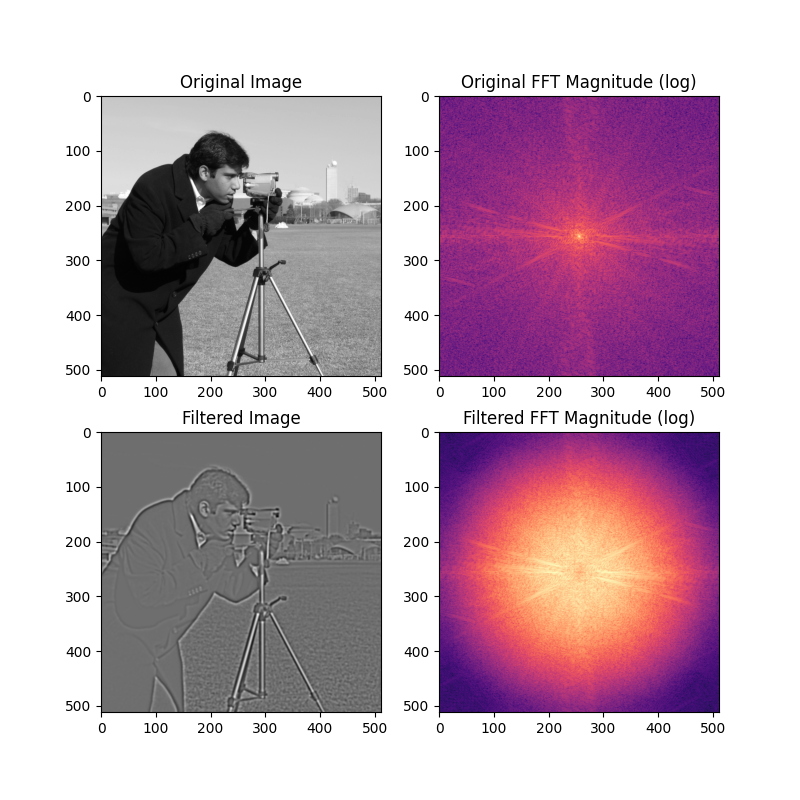# Band-pass filtering by Difference of Gaussians¶

Band-pass filters attenuate signal frequencies outside of a range (band) of interest. In image analysis, they can be used to denoise images while at the same time reducing low-frequency artifacts such a uneven illumination. Band-pass filters can be used to find image features such as blobs and edges.

One method for applying band-pass filters to images is to subtract an image blurred with a Gaussian kernel from a less-blurred image. This example shows two applications of the Difference of Gaussians approach for band-pass filtering.

## Denoise image and reduce shadows¶

```import matplotlib.pyplot as plt
import numpy as np
from skimage.data import gravel
from skimage.filters import difference_of_gaussians, window
from scipy.fft import fftn, fftshift

image = gravel()
wimage = image * window('hann', image.shape)  # window image to improve FFT
filtered_image = difference_of_gaussians(image, 1, 12)
filtered_wimage = filtered_image * window('hann', image.shape)
im_f_mag = fftshift(np.abs(fftn(wimage)))
fim_f_mag = fftshift(np.abs(fftn(filtered_wimage)))

fig, ax = plt.subplots(nrows=2, ncols=2, figsize=(8, 8))
ax[0, 0].imshow(image, cmap='gray')
ax[0, 0].set_title('Original Image')
ax[0, 1].imshow(np.log(im_f_mag), cmap='magma')
ax[0, 1].set_title('Original FFT Magnitude (log)')
ax[1, 0].imshow(filtered_image, cmap='gray')
ax[1, 0].set_title('Filtered Image')
ax[1, 1].imshow(np.log(fim_f_mag), cmap='magma')
ax[1, 1].set_title('Filtered FFT Magnitude (log)')
plt.show()
```## Enhance edges in an image¶

```from skimage.data import camera

image = camera()
wimage = image * window('hann', image.shape)  # window image to improve FFT
filtered_image = difference_of_gaussians(image, 1.5)
filtered_wimage = filtered_image * window('hann', image.shape)
im_f_mag = fftshift(np.abs(fftn(wimage)))
fim_f_mag = fftshift(np.abs(fftn(filtered_wimage)))

fig, ax = plt.subplots(nrows=2, ncols=2, figsize=(8, 8))
ax[0, 0].imshow(image, cmap='gray')
ax[0, 0].set_title('Original Image')
ax[0, 1].imshow(np.log(im_f_mag), cmap='magma')
ax[0, 1].set_title('Original FFT Magnitude (log)')
ax[1, 0].imshow(filtered_image, cmap='gray')
ax[1, 0].set_title('Filtered Image')
ax[1, 1].imshow(np.log(fim_f_mag), cmap='magma')
ax[1, 1].set_title('Filtered FFT Magnitude (log)')
plt.show()
```Total running time of the script: ( 0 minutes 0.570 seconds)

Gallery generated by Sphinx-Gallery